# An Introduction to Graph Neural NetworksImage Credits

Deep Learning is suitable for capturing hidden patterns of Euclidean data like images (2D grids) and texts (1D sequences). But what about applications where data is generated from non-Euclidean domains, depicted as graphs with complicated relationships and interdependencies between entities?

That’s where Graph Neural Networks (GNN) come in

In this post, we will learn about graph theories a, GNN forms and principles, and GNNs applications.

The term GNN is typically referred to a combination of diverse algorithms and not a single architecture. As we will see, a superabundance of diverse architectures has been developed over the years. To give you an early preview, here is a diagram illustrating the most important papers in the field.

The diagram has been borrowed from a recent review paperon GNNs by Zhou J. et al.Different types of Graph Neural Networks. Credits

A graph is a data structure consisting of two components: nodes (vertices) and edges.

G = (V, E), where V is the set of nodes, and E are the edges that define the relationship between nodes.

If there are directional dependencies between nodes then edges are directed. If not, edges are undirected.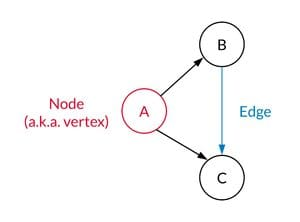Directed Graph with nodes and edges. Credits

A graph may be used to describe various objects, including social media networks, city networks, compounds, and molecules. A simple graph might look like this below: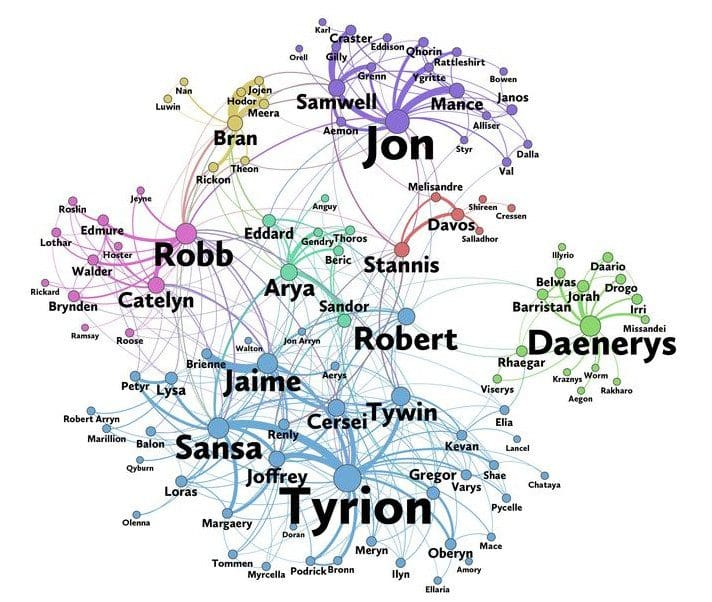A network graph of the characters in the Game of Thrones. Credits

Graph Neural Networks are a specific type of neural networks that are capable of working with graph structures. They are influence by convolutional neural networks to perform graph and node classification, link prediction,and community detection.Graph Neural Networks. Credits.

Using graph data any neural network is required to perform tasks using the vertices or nodes of the data. For example, if we are performing any classification job using any of the available GNN then the graph network is needed to classify the vertices or nodes of the graph data. In graph data, nodes should be shown with their labels so that every node can be classified by its labels according to the neural networks.

First we map nodes to a d-dimensional embedding space (low dimensional space rather than the actual dimension of the graph) using Node Embeddings. It make sure that the identical nodes in the graph are implanted close to each other.

The objective here is to map nodes so that similarity in the embedding space resembles similarity in the network.

Let’s define u and v as two nodes in a graph.

xu and xv are two feature vectors.

Next we’ll define the encoder function Enc(u) and Enc(v), which transform the feature vectors to z_u and z_vImage Credits

The encoder function should be capable of accomplishing:

• Locality (local network neighborhoods)
• Aggregate information
• Stacking multiple layers (computation)

Locality information can be accomplished by using what we call a computational graph. As shown in the graph below, i is the red node where we see how this node is linked to its neighbors and those neighbors’ neighbors. We’ll see all the possible links, and form a computation graph.

By doing this, we’re grasping the graph skeleton, and also borrowing feature information at the same time.Neighborhood exploration and information sharing. Credits

Once the locality information holds the computational graph, we start the aggregating process. This is essentially done using neural network architecture.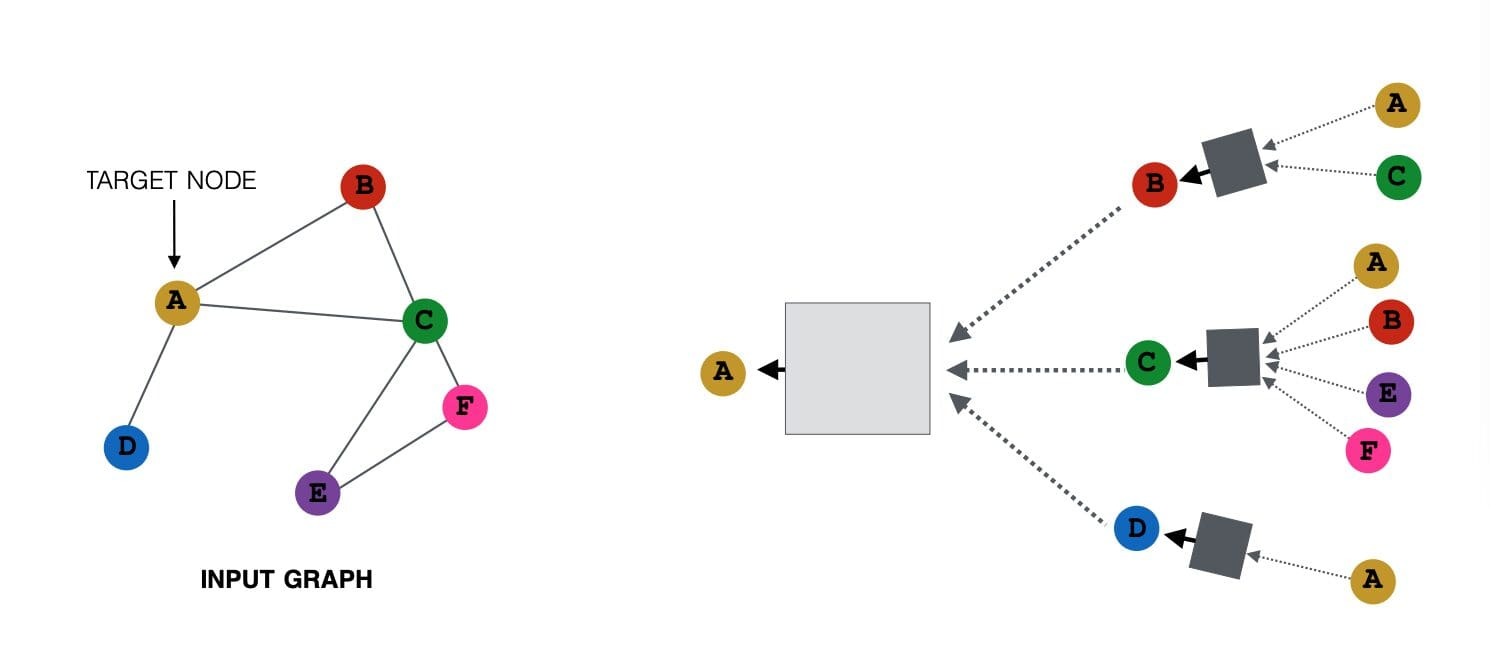Consider the above image, On the right is the input graph and a target node A, the graph on the right side shows the computation graph of node A based on its neighborhood. Intuitively, node A acquires all the messages from its neighborhood nodes [B, C, D] and transforms them, and the nodes [B, C, D] in turn transform information from their neighbors. Take a look at the edge directions to comprehend the computational graph. This is a computational graph of depth 2, where original node features of nodes [A, C] are passed to node B, transformed, and then passed again to node A.

The essential intuition is that every node has its own computational graph, and we make use of dynamic recursive programming, and simultaneously calculate node embeddings of all the nodes at each layer of the computational graph and provide them into the next layer of the computational graph.Every node has a feature vector.

For instance, (X_A) is a feature vector of node A.

The inputs are those feature vectors, and the box will take the two feature vectors (X_A and X_C), aggregate them, and then pass them on to the next layer.

So in order to perform forward propagation in this computational graph, we require 3 steps:

1. Initialize the activation units: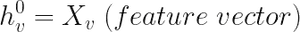1. Every layer in the network: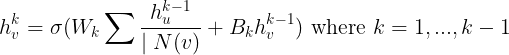We can notice that there are two parts to this equation:

• The first part is averaging all the neighbors of node v.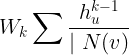• The second part is the previous layer embedding of node v multiplied with a bias Bk, which is a trainable weight matrix and it’s basically a self-loop activation for node v.• The non-linearity activation σ is performed on the two parts.
1. At the final layer:It’s the embedding after K layers of neighborhood aggregation.

Training the Model

We can provide the embeddings into any loss function and run stochastic gradient descent to train the weight parameters. For example, for a binary classification job, we can define the loss function as: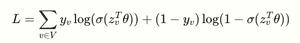Where,

y_v ∈ {0,1} is the node class label.

z_v is the encoder output.

θ is the classification weight.

σ can be the sigmoid function.

σ(z_v^Tθ represents the predicted probability of node v.

If the label is positive (y_v=1) the first half of equation would contribute to loss function, else the second half of equation would contribute to the loss function.

Training can be unsupervised or supervised:

Unsupervised training:

• Here we use only the graph layout: similar nodes have similar embeddings. An unsupervised loss function can be a loss based on node nearness in the graph, or random walks.

Supervised training:

• Train model for node classification, normal or anomalous node.

This is the crux behind the amazing power of Graph Neural Networks. How we aggregate the messages at each level, and how we calculate the message from a node, using all the node neighborhood information are the riffs on our Graph Neural Network.

Look around, graphs are all around us, be it the real world or our engineered systems. More often than not, the data we see in machine learning problems is structured or relational, and thus can also be described with a graph. And while fundamental research on GNNs is perhaps decades old, recent advancements in the capabilities of modern GNNs have led to advances in professions as varied as traffic prediction,rumor, and fake news detection, modeling disease spread, physics simulations, and understanding why molecules smell.

In Natural Language Process, GNNs are used to understand the relation between word and context. It is hard to understand context using structure text data even with large language models. If you want the machine learning models to succeed in customer face application, then you must start investing on graph and GNNs.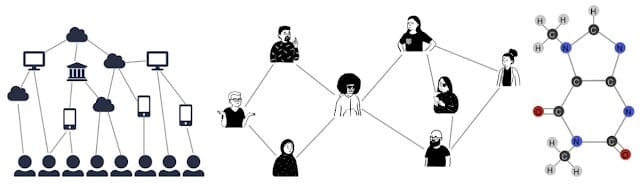Graphs can model the relationships between many different types of data, including web pages (left), social connections (center), or molecules (right). Credits

Graphs are hard to visualize for humans. It does not exist in 2D or 3D space. A graph is dynamic as it does not hold a fixed shape. This makes it harder for you to analyze the data using traditional tools. The large size and complexity add another layer of difficulty. It will be hard to extract insight if your graph structure is dense.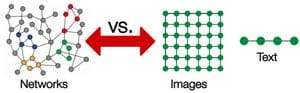CNNs can be used to make machines envision things, and perform tasks like image classification, image recognition, or object detection. This is where CNNs are the most prevalent. CNNs on graph fails due to it size and complexit of graph data. There is no fixed order of node and it changes inputs of matrix in a network.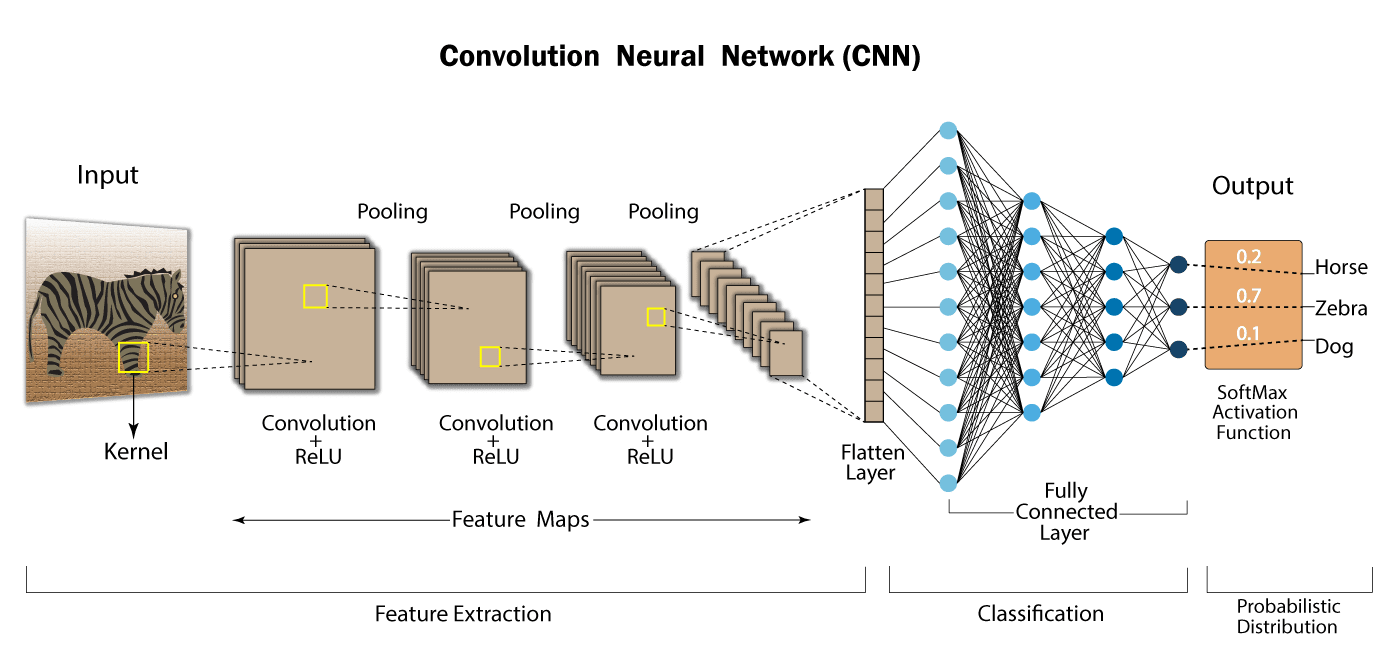CNN on an image. Credits

GNNs can be designed to make predictions at the level of nodes, edges, or entire graphs:

• Node Classification: we use neighboring nodes to predict missingnode labels using CNNs
• Link Prediction: it is commonly used of social networks to predict missing links between nodes.
• Graph Classification:it is using in text classification where we classify graphs into various categories.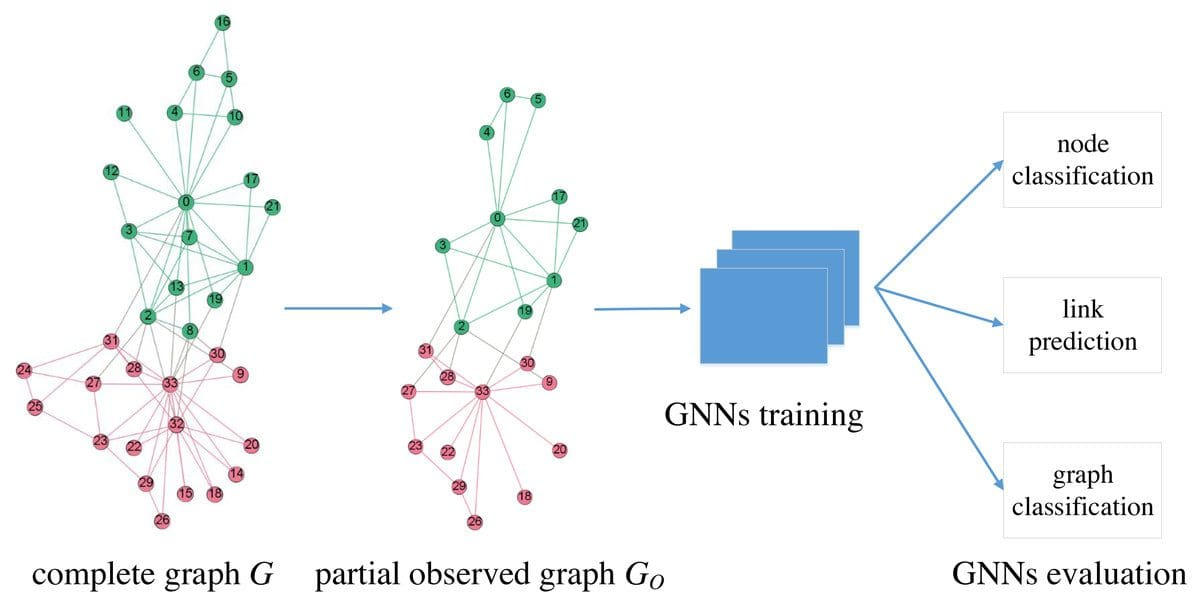Image Credits

Graph neural networks are very powerful tools to solve complex data problems. If you want to training you own graph model, check out Goggle Colab with video tutorials to learn about PyTorch Geometric adn how you can use GNNs to build machine learning applications.

Thank you for reading!

## References

https://petar-v.com/talks/SOM-GNN.pdf

Graph Neural Network and Some of GNN Applications: Everything You Need to Know

https://wandb.ai/syllogismos/machine-learning-with-graphs/reports/8-Graph-Neural-Networks–VmlldzozNzcwMTA

https://blog.tensorflow.org/2021/11/introducing-tensorflow-gnn.html

Nagesh Singh Chauhan holds a Bachelor’s degree in Computer Science and currently works as Senior Manager-Data Science at OYO (the world’s leading chain of hotels and homes). Nagesh has specializations in various domains related to data science, including machine learning, deep learning, NLP, time-series analysis, probability and statistics, computer vision, big data, and embedded systems. Nagesh also loves to write technical articles on various aspects of AI and data science. You can check out some of his articles on theaidream and Github. You can also reach out on LinkedIn.